Folder Contest Winners ( 2 sub folders ) 12 Ahuizotl 4 Anthro 0 Discord 8 Dragons 7 Equestria Girls 28 Griffons 11 Humans 7 Iron Will 6 Lord Tirek 9 Mature 13 Ponies and Hands 13

Welcome to Soft Hands, the home of all things Soft Hands!H E Y C H E C K O U T M Y S O F T H A N D S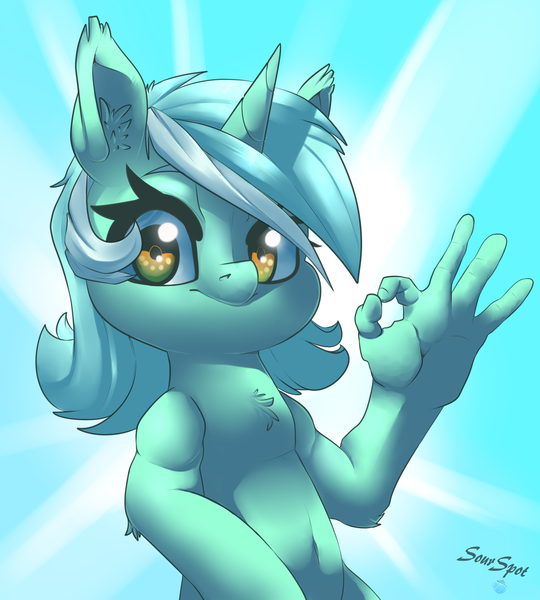Y O U S A I D S O F T H A N D S A L L T H E T I M E G E T S O M E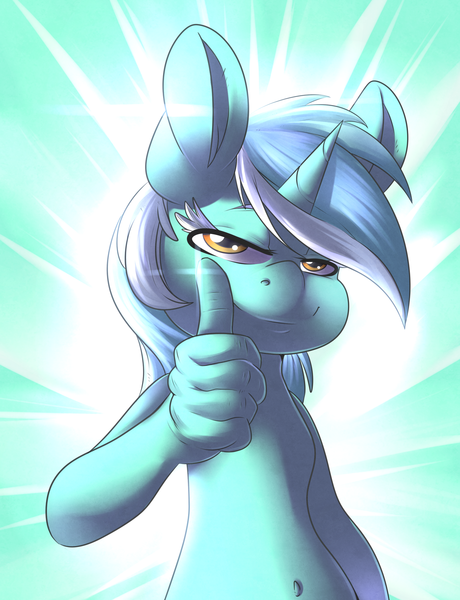I H A V E S O F T H A N D S I W E A R S O F T H A N D S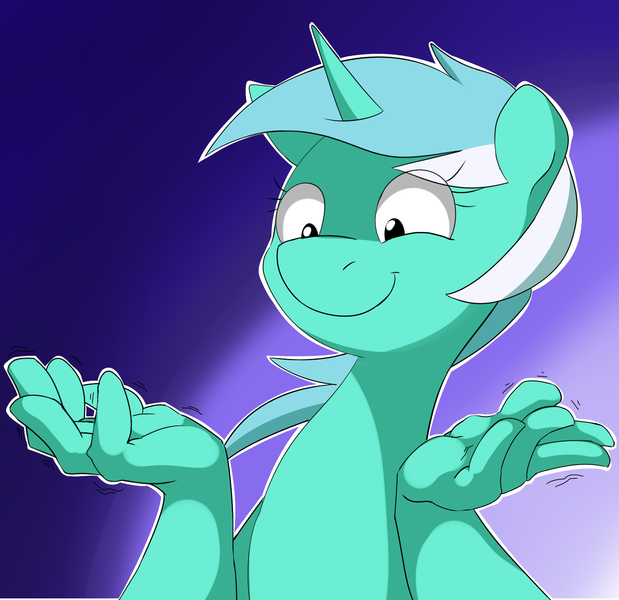I M A S O F T H A N D S M A N S O F T H A N D S S O F T H A N D SS O F T H A N D S T H E S O F T E S T H A N D S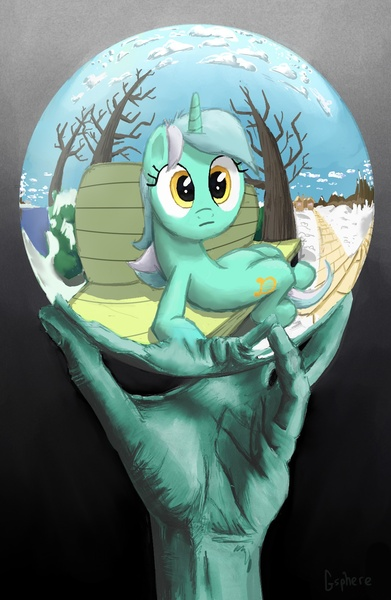E V E R Y B O D Y E V E R Y B O D Y S O F T E S T H A N D S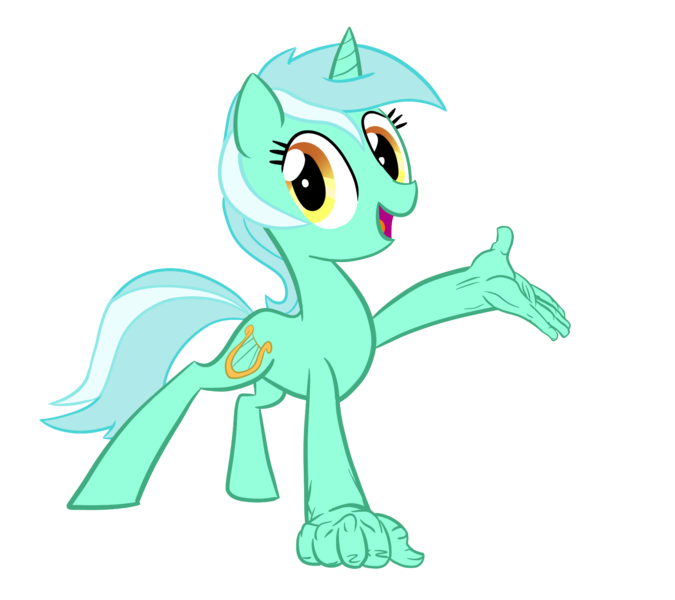S O F T S O F T S O F T S O F T S O F T S O F T H A N D S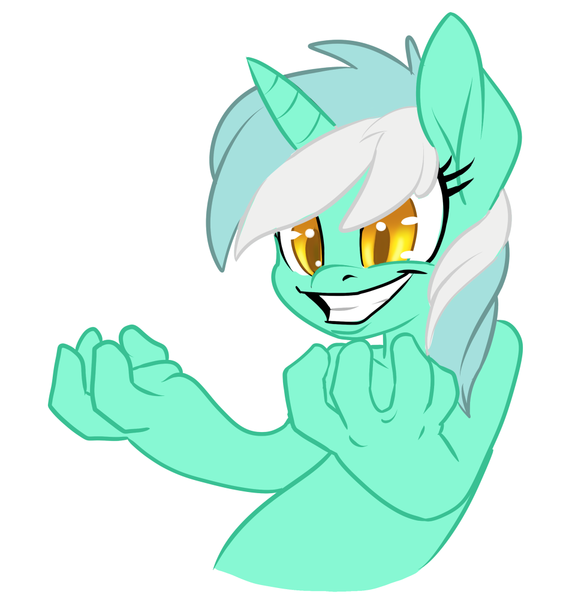I W A S T O L D S O F T H A N D S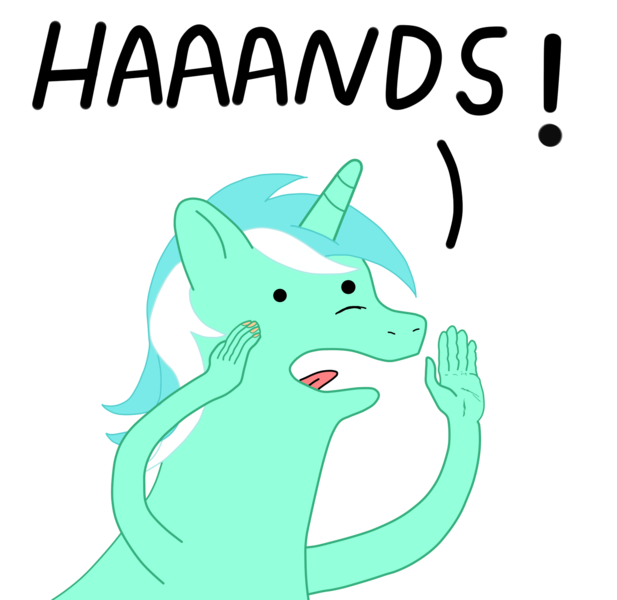T H E Y S A I D S O F T H A N D S A L W A Y S S O F T H A N D S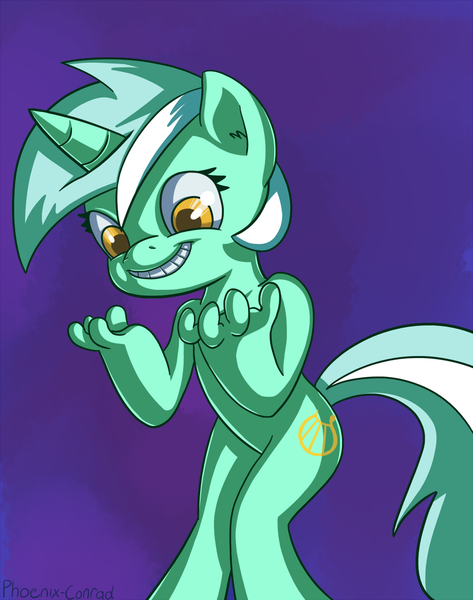S O F T M Y H A N D S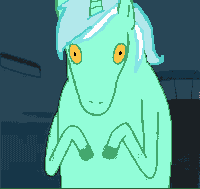G L E A M I N G H A N D S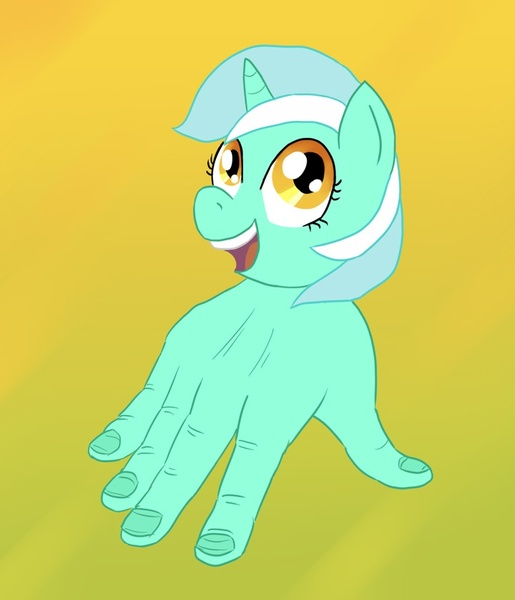G L O R I O U S H A N D SN O F E E T N O F E E T N O F E E T N O F E E T N O F E E T N O F E E T N O F E E T N O F E E T